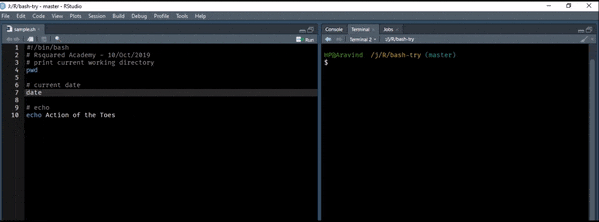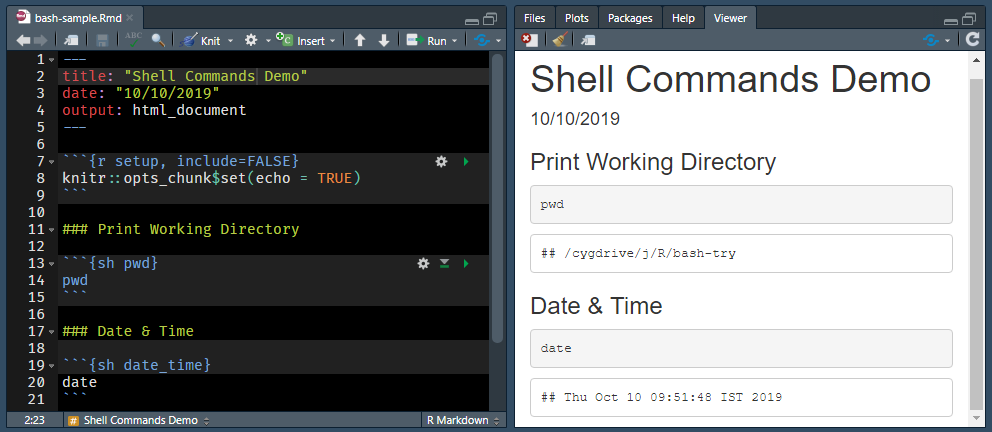# Chapter 10 R & the Shell

In this chapter, we will learn to

• execute shell commands from R
• execute shell script in RStudio
• execute shell commands in RMarkdown
• execute R commands & script in the shell

## 10.1 R

Now, let us turn our attention to executing commands from R using `system2()`. Here we will focus on the following

• execute a command without arguments
• execute commands with arguments
• redirect output

Let us try to execute a command without any additional arguments. We will execute the `ls` command to list all files and directories. Use `system2()` and specify the command using the `command` argument. Whenever you are trying to execute a command from R, the first argument or input should be the command and it must be enclosed in quotes.

``system2(command = "ls")``

Great! Now, how do we specify the options? The additional options of a command must be stored as a character vector and specified using the `args` argument. In the below example, we delete the `examples` folder we created earlier while decompressing the `zip_example.zip` file.

``````system2(command = "rm",
args    = c("-r", "examples"))``````

In some cases, we might want to redirect the output. Let us say we are writing message to a file using the `echo` command. In this cases, we want the output to be redirected to the `release.txt` file. The `stdout` argument can be used to redirect output to a file or the R console. In the below example, we redirect the output to a file.

``````system2(command = "echo",
args    = c("Great Truth"),
stdout  = "release.txt")``````

In the next example, we redirect the output to the R console by setting the value of the `stdout` argument to `TRUE`. If you are curious, set the value to `FALSE` and see what happens.

``````system2(command = "diff",
args    = c("imports_olsrr.txt", "imports_blorr.txt"),
stdout  = TRUE)``````

The `run()` command from the processx package can be used to execute shell commands as well.

## 10.2 RStudio

In RStudio, commands can be executed from shell scripts by pressing `Ctrl + Enter`. Instead of sending the command to the R console, it is redirected to the terminal where it is executed as shown below.## 10.3 RMarkdown

RMarkdown supports `bash`, `sh` and `awk`. This post was initially created using `sh` as the underlying operating system is Windows. Later, we used `bash` after installing the Windows subsystem for Linux. Click here to learn more.## 10.4 Rscript

In this section, we will learn to execute R commands and scripts in the command line using:

• `R -e`
• `Rscript -e`
• `R CMD BATCH`

The `-e` option allows us to specify R expression(s). `R -e` will launch R and then execute the code specified within quotes. Use semi-colon to execute multiple expressions as shown below. You will be able to run the below commands only if you are able to launch R from the command line. Windows users need to ensure that R is added to the path environment.

``R -e "head(mtcars); tail(mtcars)"``

`Rscript -e` will run code without launching R.

``Rscript -e "head(mtcars)"``

We can use `Rscript` to execute a R script as well. In the below example, we execute the code in `analysis.R` file.

``Rscript analysis.R``

If you are more interested, try the littler package as well.